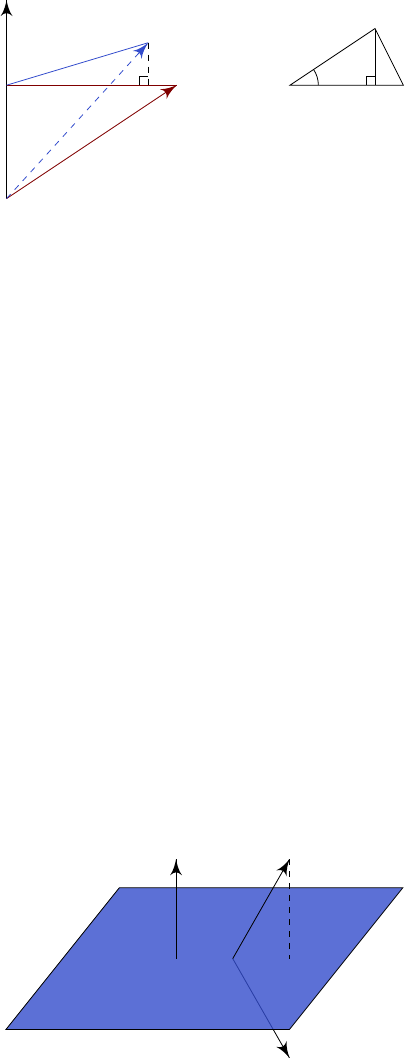3Linear maps

IA Vectors and Matrices3.1 Examples
3.1.1 Rotation in R
3
In
R
3
, first consider the simple cases where we rotate about the
z
axis by
θ
. We
call this rotation R and write x
0
= R(x).
Suppose that initially,
x
= (
x, y, z
) = (
r cos φ, r sin φ, z
). Then after a
rotation by θ, we get
x
0
= (r cos(φ + θ), r sin(φ + θ), z)
= (r cos φ cos θ r sin φ sin θ, r sin φ cos θ + r cos φ sin θ, z)
= (x cos θ y sin θ, x sin θ + y cos θ, z).
We can represent this by a matrix
R
such that
x
0
i
=
R
ij
x
j
. Using our formula
above, we obtain
R =
cos θ sin θ 0
sin θ cos θ 0
0 0 1
Now consider the general case where we rotate by θ about
ˆ
n.
O
ˆ
n
A
x
B
A
0
C
x
0
B A
A
0
C
θ
We have x
0
=
OB +
BC +
CA
0
. We know that
OB = (ˆn · x)ˆn
BC =
BA cos θ
= (
BO +
OA) cos θ
= ((ˆn · x)ˆn + x) cos θ
Finally, to get
CA
, we know that
|
CA
0
|
=
|
BA
0
|sin θ
=
|
BA|sin θ
=
|ˆn × x|sin θ
.
Also,
CA
0
is parallel to
ˆ
n × x. So we must have
CA
0
= (
ˆ
n × x) sin θ.
Thus x
0
= x cos θ + (1 cos θ)(ˆn · x)ˆn + ˆn × x sin θ. In components,
x
0
i
= x
i
cos θ + (1 cos θ)n
j
x
j
n
i
ε
ijk
x
j
n
k
sin θ.
We want to find an R such that x
0
i
= R
ij
x
j
. So
R
ij
= δ
ij
cos θ + (1 cos θ)n
i
n
j
ε
ijk
n
k
sin θ.
3.1.2 Reflection in R
3
Suppose we want to reflect through a plane through
O
with normal
ˆ
n
. First of
all the projection of
x
onto
ˆ
n
is given by (
x ·
ˆ
n
)
ˆ
n
. So we get
x
0
=
x
2
(x · ˆn)ˆn
.
In suffix notation, we have
x
0
i
=
x
i
2
x
j
n
j
n
i
. So our reflection matrix is
R
ij
= δ
ij
2n
i
n
j
.
x
0
ˆ
n x